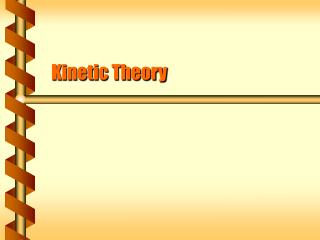DownloadDownload PresentationKinetic Theory

# Kinetic Theory

Download Presentation## Kinetic Theory

- - - - - - - - - - - - - - - - - - - - - - - - - - - E N D - - - - - - - - - - - - - - - - - - - - - - - - - - -
##### Presentation Transcript

1. Kinetic Theory

2. Microscopic Analysis • The behavior of a gas should be described by the molecules. • The gas consists of a large number of identical particles of mass m. • The particles have negligible size and no internal structure. • The particles are moving in random dirctions with speeds independent of direction • Collisions between particles and with the walls are perfectly elastic.

3. Collision Force • Follow a collision with a wall perpendicular to x. • Particle has mass m and a velocity vx. • Strike the wall: Dt = 2L/vx. • Impulse: Dp = 2mvx. • The force from one particle: L Fx v m

4. Pressure Force • The pressure on the wall comes from all the particles. • The volume is V = AL. • Find the value for N particles. L A

5. Three Dimensions • The pressure come from all three dimensions, and is equal in all three. • Relate the pressure to the average speed. V P N

6. Theory Match • The expression from a particle level relates the average kinetic energy. • This almost matches the ideal gas law. V P N

7. Temperature as Energy • For the particle-level theory to match the experimental law we equate them. • This is kinetic theory. • Temperature measures the average kinetic energy.

8. What is the average energy of an air molecule at room temperature (293 K)? What is average speed for a nitrogen molecule (28 g/mol)? Energy directly relates to temperature. (3/2)kT = 6.07 x 10-21 J. To get the speed requires the mass. m = (0.028 kg/mol) / (6.022 x 1023 /mol) = 4.65x10-26 kg Average Energy

9. Distribution of Speeds • Kinetic theory used the average speed. • Actual atoms fall into a range of speeds. • The Maxwell-Boltzmann distribution describes the probability of a molecule having a particular speed.

10. Real Gases • The assumptions for kinetic theory are approximately true. • Non-zero molecular size • Non-zero force between molucules • For the Van der Waals force in air, the effect is about 1% difference from an ideal gas. next